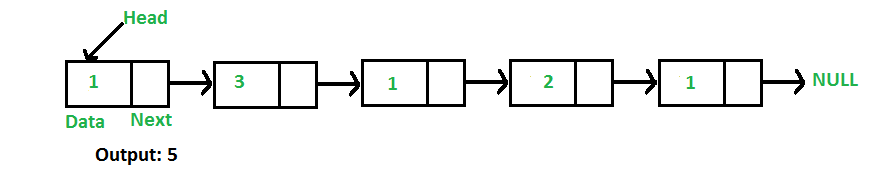# C++ Program For Finding Length Of A Linked List

Write a function to count the number of nodes in a given singly linked list.For example, the function should return 5 for linked list 1->3->1->2->1.

Iterative Solution:

```1) Initialize count as 0
2) Initialize a node pointer, current = head.
3) Do following while current is not NULL
a) current = current -> next
b) count++;
4) Return count ```

Following is the Iterative implementation of the above algorithm to find the count of nodes in a given singly linked list.

## C++

 `// Iterative C++ program to find length ` `// or count of nodes in a linked list ` `#include ` `using` `namespace` `std;`   `// Link list node` `class` `Node ` `{ ` `    ``public``:` `    ``int` `data; ` `    ``Node* next; ` `}; `   `/* Given a reference (pointer to pointer)` `   ``to the head of a list and an int, push ` `   ``a new node on the front of the list. */` `void` `push(Node** head_ref, ``int` `new_data) ` `{ ` `    ``// Allocate node` `    ``Node* new_node =``new` `Node();`   `    ``// Put in the data` `    ``new_node->data = new_data; `   `    ``// Link the old list of the ` `    ``// new node` `    ``new_node->next = (*head_ref); `   `    ``// Move the head to point to ` `    ``// the new node` `    ``(*head_ref) = new_node; ` `} `   `// Counts no. of nodes in linked list` `int` `getCount(Node* head) ` `{ ` `    ``// Initialize count ` `    ``int` `count = 0; `   `    ``// Initialize current ` `    ``Node* current = head;` `    ``while` `(current != NULL) ` `    ``{ ` `        ``count++; ` `        ``current = current->next; ` `    ``} ` `    ``return` `count; ` `} `   `// Driver code` `int` `main() ` `{ ` `    ``// Start with the empty list` `    ``Node* head = NULL; `   `    ``// Use push() to construct list ` `    ``// 1->2->1->3->1` `    ``push(&head, 1); ` `    ``push(&head, 3); ` `    ``push(&head, 1); ` `    ``push(&head, 2); ` `    ``push(&head, 1); `   `    ``// Check the count function` `    ``cout << ``"count of nodes is "` `<< ` `             ``getCount(head); ` `    ``return` `0; ` `} `   `// This is code is contributed by rathbhupendra`

Output:

`count of nodes is 5`

Time Complexity: O(n), where n represents the length of the given linked list.
Auxiliary Space: O(1), no extra space is required, so it is a constant.

Recursive Solution:

```int getCount(head)
1) If head is NULL, return 0.
2) Else return 1 + getCount(head->next) ```

Following is the Recursive implementation of the above algorithm to find the count of nodes in a given singly linked list.

## C++

 `// Recursive C++ program to find length` `// or count of nodes in a linked list` `#include ` `using` `namespace` `std;`   `// Link list node` `class` `Node ` `{` `    ``public``:` `    ``int` `data;` `    ``Node* next;` `};`   `/* Given a reference (pointer to pointer) ` `   ``to the head of a list and an int, ` `   ``push a new node on the front of the list. */` `void` `push(Node** head_ref, ``int` `new_data)` `{` `    ``// Allocate node ` `    ``Node* new_node = ``new` `Node();`   `    ``// Put in the data` `    ``new_node->data = new_data;`   `    ``// Link the old list of the ` `    ``// new node ` `    ``new_node->next = (*head_ref);`   `    ``// Move the head to point to ` `    ``// the new node` `    ``(*head_ref) = new_node;` `}`   `// Recursively count number of ` `// nodes in linked list ` `int` `getCount(Node* head)` `{` `    ``// Base Case` `    ``if` `(head == NULL) ` `    ``{` `        ``return` `0;` `    ``}` `    ``// Count this node plus the rest ` `    ``// of the list` `    ``else` `    ``{` `        ``return` `1 + getCount(head->next);` `    ``}` `}`   `// Driver code` `int` `main()` `{` `    ``// Start with the empty list` `    ``Node* head = NULL;`   `    ``// Use push() to construct list` `    ``// 1->2->1->3->1` `    ``push(&head, 1);` `    ``push(&head, 3);` `    ``push(&head, 1);` `    ``push(&head, 2);` `    ``push(&head, 1);`   `    ``// Check the count function` `    ``cout << ``"Count of nodes is "` `<< ` `             ``getCount(head);` `    ``return` `0;` `}` `// This is code is contributed by rajsanghavi9`

Output:

`Count of nodes is 5`

Time Complexity: O(n), where n represents the length of the given linked list.
Auxiliary Space: O(n), for recursive stack where n represents the length of the given linked list.

Please refer complete article on Find Length of a Linked List (Iterative and Recursive) for more details!

Feeling lost in the world of random DSA topics, wasting time without progress? It's time for a change! Join our DSA course, where we'll guide you on an exciting journey to master DSA efficiently and on schedule.
Ready to dive in? Explore our Free Demo Content and join our DSA course, trusted by over 100,000 geeks!

Previous
Next